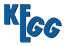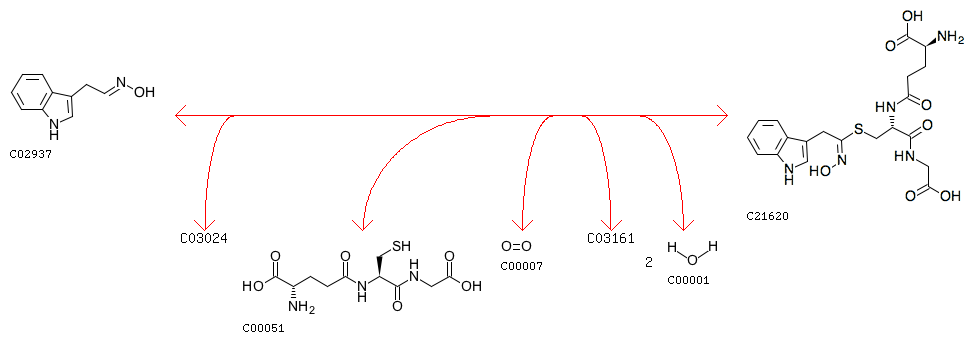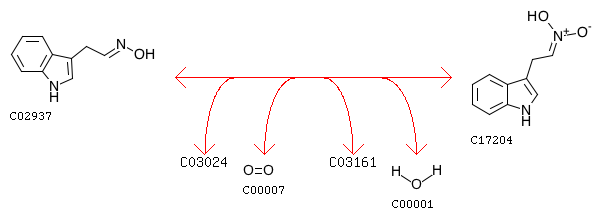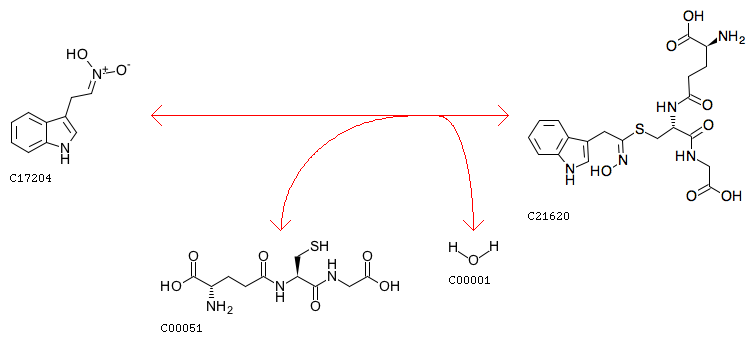REACTION: R11734
Entry
 ```R11734                      Reaction                                ```
Name
Definition
Indole-3-acetaldehyde oxime + [Reduced NADPH---hemoprotein reductase] + Glutathione + Oxygen <=> S-[(E)-N-Hydroxy(indol-3-yl)acetimidoyl]-L-glutathione + [Oxidized NADPH---hemoprotein reductase] + 2 H2O
EquationComment
two-step reaction (see R11735+R11736)
Reaction class
 C02937_C21620
Enzyme
Other DBs
 RHEA: 52183REACTION: R11735
Entry
 ```R11735                      Reaction                                ```
Definition
Indole-3-acetaldehyde oxime + [Reduced NADPH---hemoprotein reductase] + Oxygen <=> Indole-3-acetaldoxime N-oxide + [Oxidized NADPH---hemoprotein reductase] + H2O
EquationComment
the first step of two-step reaction (see R11734, R11735+R11736)
Reaction class
 C02937_C17204
Enzyme
Other DBs
 RHEA: 52187REACTION: R11736
Entry
 ```R11736                      Reaction                                ```
Definition
Indole-3-acetaldoxime N-oxide + Glutathione <=> S-[(E)-N-Hydroxy(indol-3-yl)acetimidoyl]-L-glutathione + H2O
EquationComment
spontaneous
the second step of two-step reaction (see R11734, R11735+R11736)
Reaction class
 C17204_C21620
Enzyme
Other DBs
 RHEA: 52191